# Square ABCD

Construct a square ABCD with cente S [3,2] and the side a = 4 cm. Point A lies on the x-axis. Construct square image in the displacement given by oriented segment SS'; S` [-1 - 4].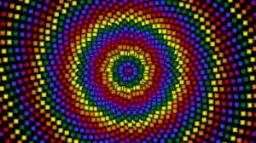Did you find an error or inaccuracy? Feel free to write us. Thank you!#### You need to know the following knowledge to solve this word math problem:

We encourage you to watch this tutorial video on this math problem:

## Related math problems and questions:

• Mrak - cloud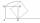It is given segment AB of length 12 cm, where one side of the square MRAK laid on it. MRAK's side length 2 cm shown. MRAK gradually flips along the line segment AB the point R leaves a paper trail. Draw the whole track of point R until square can do the l
• Rhombus constructionConstruct ABCD rhombus if its diagonal AC=9 cm and side AB = 6 cm. Inscribe a circle in it touching all sides...
• Construction of trapezoidConstruct a trapezoid if b = 4cm, c = 7cm, d = 4,5cm, v = 3 cm (Procedure, discussion, sketch, analysis, construction)
• ABCD squareIn the ABCD square, the X point lies on the diagonal AC. The length of the XC is three times the length of the AX segment. Point S is the center of the AB side. The length of the AB side is 1 cm. What is the length of the XS segment?
• Construct rhombusConstruct rhombus ABCD if given diagonal length | AC | = 8cm, inscribed circle radius r = 1.5cm
• Construct 1Construct a triangle ABC, a = 7 cm, b = 9 cm with right angle at C, construct the axis of all three sides. Measure the length of side c (and write).
• Rhombus EFGHConstruct the rhombus EFGH where e = 6.7cm, height to side h: vh = 5cm
• Sides od triangleSides of the triangle ABC has length 4 cm, 5 cm and 7 cm. Construct triangle A'B'C' that are similar to triangle ABC which has a circumference of 12 cm.
• Circle tangentIt is given to a circle with the center S and radius 3.5 cm. Distance from the center to line p is 6 cm. Construct a circle tangent n which is perpendicular to the line p.
• Draw a trapezoidDraw a trapezoid if given a = 7 cm, b = 4 cm, c = 3.5 cm, diagonal AC = 5cm. Solve as a construction task.
• Construct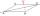Construct a rhombus ABCD, if the size of the diagonal AC is 6 cm and diagonal BD 8 cm long.
• ConstructConstruct a triangle ABC inscribed circle has a radius r = 2 cm, the angle alpha = 50 degrees = 8 cm. Make a sketch, analysis, construction and description.
• Tangents constructThe circle k is given k (S; 2.5 cm) and an outer line p. Construct a tangent t of the circle that has with a line p angle 60°. How many solutions have the task?
• Two heights and a side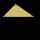Construct triangle ABC when the given side is c = 7 cm, height to side a va = 5 cm and height to side b: vb = 4 cm.
• Three points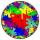Mark three points E, F and G in the plane not lie on one line. a) Draw a line segment FG b) Construct halfline (ray) EG c) Draw a line EF
• Rhombus MATHConstruct a rhombus M A T H with diagonal MT=4cm, angle MAT=120°
• Trapezoid MO-5-Z8ABCD is a trapezoid that lime segment CE is divided into a triangle and parallelogram, as shown. Point F is the midpoint of CE, DF line passes through the center of the segment BE, and the area of the triangle CDE is 3 cm2. Determine the area of the trape